# Catch up on the latest AI articles# [ArtCoder] Generate Art-like QR Code With Style Conversion!

3 main points
✔️ Using Neural Style Transfer to generate art-like QR codes
✔️ Proposed an end-to-end QR code generation method based on three loss functions
✔️ Successfully generated robust QR codes that can be read by real applications

written by Hao SuJianwei NiuXuefeng LiuQingfeng LiJi WanMingliang XuTao Ren
(Submitted on 16 Nov 2020)

Subjects: Computer Vision and Pattern Recognition (cs.CV); Multimedia (cs.MM)code：The images used in this article are from the paper, the introductory slides, or were created based on them.

## first of all

In this article, we introduce our research on generating art-like QR codes using Neural Style Transfer.

In the proposed method (ArtCoder), QR Code can be generated from a source image (Content), a target image (Style) and a message to be set, as in a general image style transformation, as follows.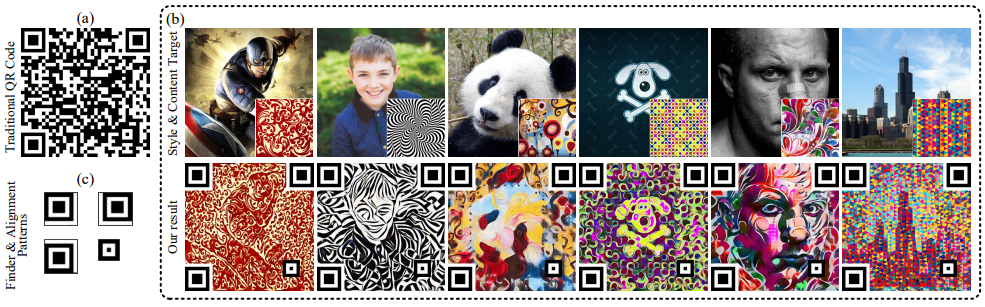If you scan this image with your smartphone or other devices, you will see the string "Thank you for reviewing our paper.

## The proposed method (ArtCoder)

### pipeline

The pipeline of the proposed methodology is as follows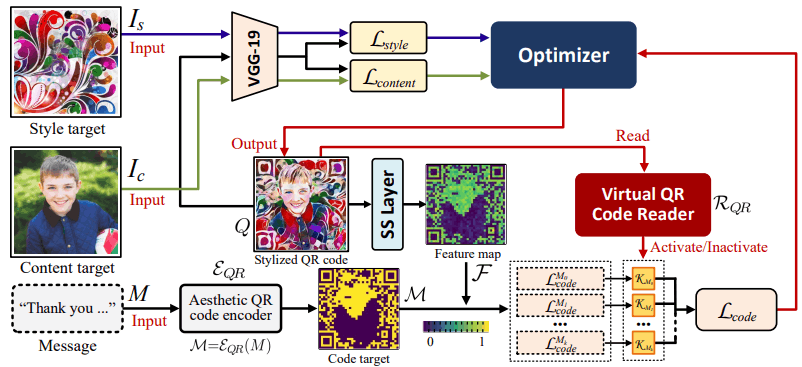The proposed method is modeled as a function $\Psi$ that generates a QR code $Q=\Psi(I_s, I_c, M)$ from a style image $I_s$, a content image $I_c$ and a message M.

In this case, the objective function (loss function) $L_{total}$ of $Q=\Psi(I_s,I_c,M)$ is defined by the following equation which combines three loss functions.

$L_{total}=\lambda_1L_{style}(I_s,Q)+\lambda_2L_{content}(I_c,Q)+\lambda_3L_code(M,Q)$

The $\lambda_1,\lambda_2,\lambda_3$ are parameters that indicate the weights of the three functions.

In addition, style loss $L_{style}$, content loss $L_{content}$, and code loss $L_{code}$ are used to preserve the style, content, and message content of QR Code, respectively. Let's look at each loss in turn below.

### Style loss $L_{style}$ and content loss $L_{content}$

The style loss $L_{style}$ and content loss $L_{content}$ are used to ensure that the generated QR Code retains its style and content contents. Specifically, according to the existing work on style transformation (1, 2), they are defined by the following equations

$L_{style}(I_s,Q)=\frac{1}{C_sH_sW_s}||G[f_s(I_s)]-G[f_s(Q)]||^2_2$

$L_{content}(I_c,Q)=\frac{1}{C_sH_sW_s}||f_c(I_c)]-f_c(Q)||^2_2$

where $G$ denotes the Gram matrix and $f_s(,f_c)$ denotes the feature map extracted from the $s(,c)$-th layer of the pre-trained VGG-19.

### About code loss $L_{code}$.

The code loss $L_{code}$ is used to control the content of the generated QR code using the SS Layer (Sampling-Simulation layer), a virtual QR code reader that simulates the sampling process of the QR code reader.

In Goole ZXing, which is used to decode QR Codes, the QR Code reader samples and decodes the center pixel of each module (the black and white squares in the QR Code).

When reading a QR code with a QR code reader, the probability $g_{M_k(i,j)}$ that a pixel existing at the coordinate $(i,j)$ with the center of each module as the origin is sampled is considered to follow the following equation.

$g_{M_k(i,j)}=\frac{1}{2\pi\sigma^2}e^{-\frac{i^2+j^2}{2\sigma^2}}$

In the Sampling-Simulation layer, based on the aforementioned equation, the sampling process when the generated QR Code is read by an actual QR Code reader is simulated to improve the robustness of the QR Code reading.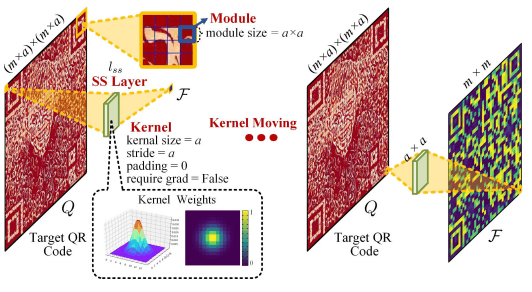Specifically, for each module $m-dis-m$ (each with $a-dis-a$ pixels) in the QR code, we perform a convolution operation with kernel size $a$, stride $a$, and padding 0, and output the feature map $F=l_ss(Q)$ of $m-dis-m$.

Based on this feature map $F=l_ss(Q)$ and $g_{M_k(i,j)}$, the bit $F_{M_k}$ corresponding to the module $M_k$ is given by

$F_{M_k}=\sum_{(i,j) \in M_k} g_{M_k(i,j)} \cdot Q_{M_k(i,j)}$

The $F_{M_k}$ is considered to simulate whether each module is decoded as 0 or 1 when the QR code is actually read by a QR code reader.

Code loss $L_{code}$

The code loss $L_{code}$ is obtained as the sum of the subcode losses $L^{M_k}_{code}$ corresponding to each module $M_k \in Q$ of QR code $Q$.

$L_{code}=\sum_{M_k in Q}L^{M_k}_{code}$

where $L^{M_k}_{code}$ is given by the following equation.

$L^{M_k}_{code}=K_{M_k} \cdot ||\textit{M}_{M_k}-F_{M_k}||$

where $\textit{M}$ is an $m×m$ matrix representing the target QR code and whether each module should be 0 or 1. Also, $K$ is the activation map computed by the competition mechanism described below.

Competition mechanism

By controlling the activation map $K$, the competition mechanism decides whether to prioritize and optimize the visual quality of the generated QR code ($L_style, L_content$) or whether the QR code can be accurately read by a QR code reader ($L_code$ ).

The pipeline for this competition mechanism is shown in the figure below.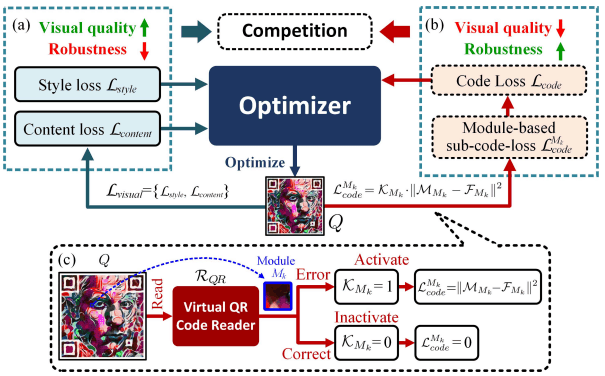Specifically, the activation map $K$ is 0 if the module $M_k$ is correct and 1 if it is wrong when the QR code $Q$ is read by the virtual QR code reader $R_{QR}$.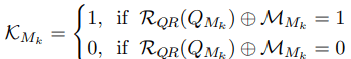By adopting such a competition mechanism, we prioritize and optimize $L_{code}$ for wrong modules and $L_{style}, L_{content}$ for correct modules, so that both image quality and robustness of QR code reading are properly and robustness of QR code reading.

About the virtual QR code reader $R_{QR}$.

When a normal QR code reader reads QR code $Q$, QR code $Q$ is converted to grayscale, and binarization is performed as follows according to the value of each module.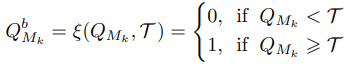Here, $T$ is the threshold for determining whether a module is black or white.

On the other hand, when reading a QR code $Q$ with a virtual QR code reader, binarization is performed for each module $M_k$ as follows.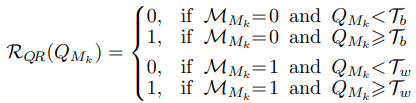## summary

In this article, we have presented our work on generating art-like QR codes using Neural Style Transfer. The proposed method (ArtCoder) is capable of generating QR codes with excellent quality and robustness that can be read by real-world applications.

However, if the generation speed is sufficiently improved in future research, we may see the day when we encounter eye-catching QR Codes with an artistic style in the real world!

If you have any suggestions for improvement of the content of the article,